GROUP THEORY SYMBOL

# GTSGCharacterTable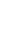GTSGCharacterTable[space group, k-point, reciprocal basis]
gives the character table of a space group at the wave vector k.

## DetailsDetails

• The algorithm is based on an induction scheme as proposed by Aroyo et al. GTSGCharacterTable calls GTSGGetIreps.
•  GOVerbose True Controls if output is printed GOGeneralPositionIreps True Controls if the character table of the full space group or the character table of the space group of k is printed.
• See:
• M. I. Aroyo et al., Acta Crystallographica Section A, A62, 115128 (2006).
• W. Hergert, M. Geilhufe, Group Theory in Solid State Physics and Photonics. Problem Solving with Mathematica, chapter 6.

## ExamplesExamplesopen allclose all

### Basic Examples  (1)Basic Examples  (1)

• First load the package.
•  In:=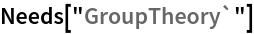• We choose the monoclinic space group P21/c (#14). We first specify the monoclinic basis vectors and calculate the corresponding basis vectors of the reciprocal lattice using GTReciprocalBasis. Then we install the space groups using GTInstallGroup.
•  In:=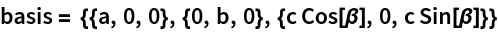Out=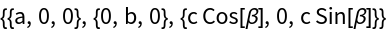In:=Out=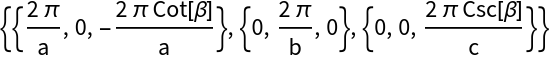In:=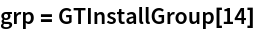Out=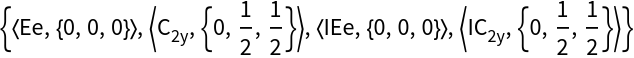In:=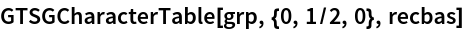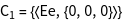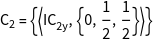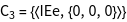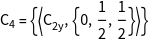Out=## See AlsoSee Also

GTCharacterTableGTSGGetIreps